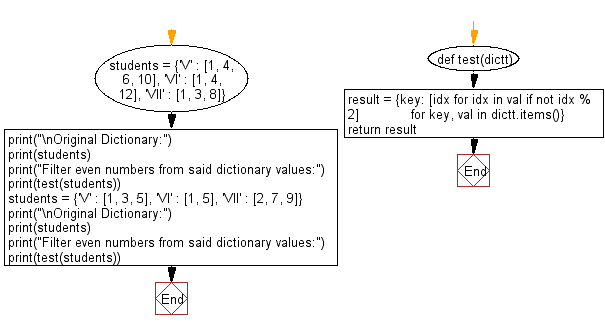﻿ Python: Filter even numbers from a given dictionary values - w3resource# Python: Filter even numbers from a given dictionary values

## Python dictionary: Exercise-57 with Solution

Write a Python program to filter even numbers from a given dictionary values.

Sample Solution:

Python Code:

``````def test(dictt):
result = {key: [idx for idx in val if not idx % 2]
for key, val in dictt.items()}
return result

students = {'V' : [1, 4, 6, 10], 'VI' : [1, 4, 12], 'VII' : [1, 3, 8]}
print("\nOriginal Dictionary:")
print(students)
print("Filter even numbers from said dictionary values:")
print(test(students))

students = {'V' : [1, 3, 5], 'VI' : [1, 5], 'VII' : [2, 7, 9]}
print("\nOriginal Dictionary:")
print(students)
print("Filter even numbers from said dictionary values:")
print(test(students))
```
```

Sample Output:

```Original Dictionary:
{'V': [1, 4, 6, 10], 'VI': [1, 4, 12], 'VII': [1, 3, 8]}
Filter even numbers from said dictionary values:
{'V': [4, 6, 10], 'VI': [4, 12], 'VII': }

Original Dictionary:
{'V': [1, 3, 5], 'VI': [1, 5], 'VII': [2, 7, 9]}
Filter even numbers from said dictionary values:
{'V': [], 'VI': [], 'VII': }
```

Pictorial Presentation:Flowchart:## Visualize Python code execution:

The following tool visualize what the computer is doing step-by-step as it executes the said program:

Python Code Editor:

Have another way to solve this solution? Contribute your code (and comments) through Disqus.

What is the difficulty level of this exercise?

Test your Programming skills with w3resource's quiz.

﻿

## Python: Tips of the Day

Clamps num within the inclusive range specified by the boundary values x and y:

Example:

```def tips_clamp_num(num,x,y):
return max(min(num, max(x, y)), min(x, y))
print(tips_clamp_num(2, 4, 6))
print(tips_clamp_num(1, -1, -6))
```

Output:

```4
-1
```